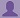SHARE
HELP

To start with, Sr2Jr’s first step is to reduce the expenses related to education. To achieve this goal Sr2Jr organized the textbook’s question and answers. Sr2Jr is community based and need your support to fill the question and answers. The question and answers posted will be available free of cost to all.# The Essentials Of Computer Organization And Architecture

Authors:
Linda Null ,julia Lobur
Exercise:
Exercises
Chapter:
Introduction
Edition:
3
ISBN:
9780763704445
Question:
2
 Previous Next

###Question

a) How many milliseconds (ms) are in 1 second?

b) How many microseconds (μs) are in 1 second?

c) How many nanoseconds (ns) are in 1 millisecond?

d) How many microseconds are in 1 millisecond?

e) How many nanoseconds are in 1 microsecond?

f) How many kilobytes (KB) are in 1 gigabyte (GB)?

g) How many kilobytes are in 1 megabyte (MB)?

h) How many megabytes are in 1 gigabyte (GB)?

i) How many bytes are in 20 megabytes?

j) How many kilobytes are in 2 gigabytes?

###Answer

a. One second is 1000 or 103 milliseconds. Milliseconds are represented as ms.
b. One second is 1000000 or 106 microseconds. Microseconds are represented as .
c. One millisecond is 1000000 or 106 nanoseconds. Nanoseconds are represented as ns.
d. One millisecond is 1000 or 103 microseconds. Microseconds are represented as .
e. One microsecond is 1000 or 103 nanoseconds. Nanoseconds are represented as ns.
f.One gigabyte (GB) is 230 kilo bytes (OR) 1 gigabyte =106 kilobytes.
g. One megabyte (MB) is 220 kilobytes (OR) 1 megabyte = 103 kilobytes.
h. One gigabyte (GB) is 210 megabytes OR 1 gigabyte = 103 megabytes
i. 20 megabytes = 20*220 bytes.
j. 2 gigabytes = 2*230 bytes.

### Discussions

Post the discussion to improve the above solution.